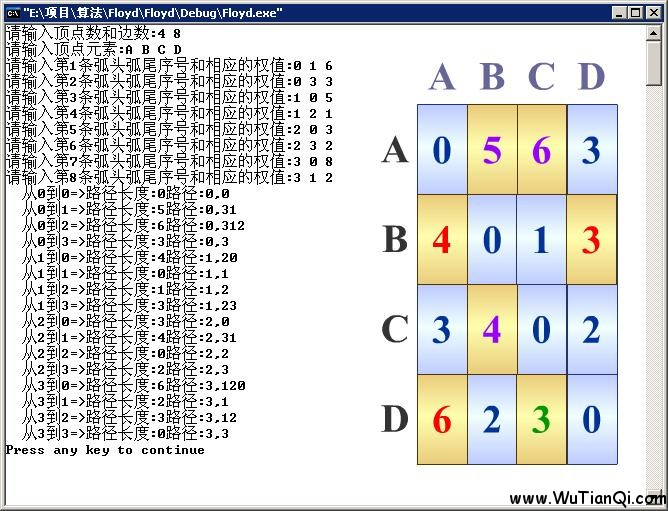# 最短路径算法—Floyd(弗洛伊德)算法分析与实现(C/C++)

17 Jan 2011

Floyd-Warshall算法，简称Floyd算法，用于求解任意两点间的最短距离，时间复杂度为O(n^3)。

Floyd-Warshall 算法用来找出每对点之间的最短距离。它需要用邻接矩阵来储存边，这个算法通过考虑最佳子路径来得到最佳路径。

1.注意单独一条边的路径也不一定是最佳路径。 2.从任意一条单边路径开始。所有两点之间的距离是边的权，或者无穷大，如果两点之间没有边相连。 对于每一对顶点 u 和 v，看看是否存在一个顶点 w 使得从 u 到 w 再到 v 比己知的路径更短。如果是更新它。 3.不可思议的是，只要按排适当，就能得到结果。 伪代码:

``````// dist(i,j) 为从节点i到节点j的最短距离
For i←1 to n do
For j←1 to n do
dist(i,j) = weight(i,j)

For k←1 to n do // k为“媒介节点”
For i←1 to n do
For j←1 to n do
if (dist(i,k) + dist(k,j) < dist(i,j)) then // 是否是更短的路径？
dist(i,j) = dist(i,k) + dist(k,j)
``````

``````void Floyd(){
int i,j,k;
for(k=1;k<=n;k++)
for(i=1;i<=n;i++)
for(j=1;j<=n;j++)
if(dist[i][k]+dist[k][j]动画演示Floyd算法】。

1、A[][]数组初始化为各顶点间的原本距离，最后存储各顶点间的最短距离。

2、path[][]数组保存最短路径，与当前迭代的次数有关。初始化都为-1，表示没有中间顶点。在求A[i][j]过程中，path[i][j]存放从顶点vi到顶点vj的中间顶点编号不大于k的最短路径上前一个结点的编号。在算法结束时，由二维数组path的值回溯，可以得到从顶点vi到顶点vj的最短路径。

[![](https://images.tankywoo.com/oldblog/2011/01/floyd1.jpg)](https://images.tankywoo.com/oldblog/2011/01/floyd1.jpg)

```cpp

#include
#include
#include
using namespace std;
#define MaxVertexNum 100
#define INF 32767
typedef struct
{
char vertex[MaxVertexNum];
int edges[MaxVertexNum][MaxVertexNum];
int n,e;
}MGraph;

void CreateMGraph(MGraph &G;)
{
int i,j,k,p;
cout<<"请输入顶点数和边数:";
cin>>G.n>>G.e;
cout<<"请输入顶点元素:";
for (i=0;i>G.vertex[i];
}
for (i=0;i>i>>j>>p;
G.edges[i][j]=p;
}
}
void Dispath(int A[][MaxVertexNum],int path[][MaxVertexNum],int n);

void Floyd(MGraph G)
{
int A[MaxVertexNum][MaxVertexNum],path[MaxVertexNum][MaxVertexNum];
int i,j,k;
for (i=0;iA[i][k]+A[k][j])
{
A[i][j]=A[i][k]+A[k][j];
path[i][j]=k;
}
}
}
}
Dispath(A,path,G.n);
}

void Ppath(int path[][MaxVertexNum],int i,int j)
{
int k;
k=path[i][j];
if (k==-1)
{
return;
}
Ppath(path,i,k);
printf("%d,",k);
Ppath(path,k,j);
}

void Dispath(int A[][MaxVertexNum],int path[][MaxVertexNum],int n)
{
int i,j;
for (i=0;i路径长度:%d路径:",i,j,A[i][j]);
printf("%d,",i);
Ppath(path,i,j);
printf("%d\n",j);
}
}
}
}

int main()
{
freopen("input2.txt", "r", stdin);
MGraph G;
CreateMGraph(G);
Floyd(G);
return 0;
}
``````